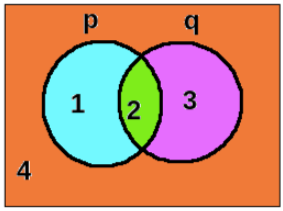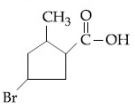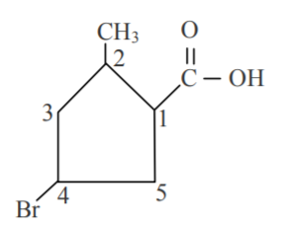Filter By

## All Questions

#### Ifis false, then the truth values of p and q are respectively :   Option: 1 F, TOption: 2 T, F Option: 3 F, F Option: 4 T, T

Relation Between Set Notation and Truth Table -

Sets can be used to identify basic logical structures of statements. Statements have two fundamental roles either it is true or false.

Let us understand with an example of two sets p{1,2} and q{2,3}.Using this relation we get-

Practise Session - 2 -

Q1. Write the truth table for the following statement pattern:-Correct Option (4)

#### The mean age of 25 teachers in a school is 40 years.  A teacher retires at the age of 60 years and a new teacher is appointed in his place.  If now the mean age of the teachers in this school is 39 years, then the age (in years) of the newly appointed teacher is : Option: 1 25 Option: 2 30 Option: 3 35 Option: 4 40

mean age   years

years

= sum of ages

new teacher be of age  let the

now

## Crack CUET with india's "Best Teachers"

• HD Video Lectures
• Unlimited Mock Tests
• Faculty Support#### Which one of the following is a tautology ?Option: 1Option: 2Option: 3Option: 4Tautology

A compound statement is called tautology if it is always true for all possible truth values of its component statement.

For example,    ( p ⇒ q ) ∨ ( q ⇒ p )

A compound statement is called a contradiction if it is always false for all possible truth values of its component statement.

For example, ∼( p ⇒ q ) ∨ ( q ⇒ p )

Truth TableQuantifiers

Quantifiers are phrases like ‘These exist’ and “for every”. We come across many mathematical statements containing these phrases.

For example –

p : For every prime number x, √x is an irrational number.

q : There exists a triangle whose all sides are equal.

-

Practise Session - 2 -

Q1. Write the truth table for the following statement pattern:-Correct Option (1)

#### The         logical         statementis equivalent to : Option: 1Option: 2Option: 3Option: 4Practise Session - 2 -

Q1. Write the truth table for the following statement pattern:-which is equal toCorrect Option (1)

## Crack NEET with "AI Coach"

• HD Video Lectures
• Unlimited Mock Tests
• Faculty Support#### Which of the following statements is a tautology ?Option: 1Option: 2Option: 3Option: 4Tautology

A compound statement is called tautology if it is always true for all possible truth values of its component statement.

For example,    ( p ⇒ q ) ∨ ( q ⇒ p )

A compound statement is called a contradiction if it is always false for all possible truth values of its component statement.

For example, ∼( p ⇒ q ) ∨ ( q ⇒ p )

Truth TableQuantifiers

Quantifiers are phrases like ‘These exist’ and “for every”. We come across many mathematical statements containing these phrases.

For example –

p : For every prime number x, √x is an irrational number.

q : There exists a triangle whose all sides are equal.

-

Practise Session - 1 -

Q1. Write the negations of the following:
1. Ram is a doctor or peon.
2. Room is clean and big.
3.is a rational number.
4. If Ram is a doctor then he is smart

-Correct Option (4)

#### Let A,B,C and D be four non-empty sets. The contrapositive statement of '' Ifandthenis :Option: 1 If, thenorOption: 2 IfthenandOption: 3 If, thenandOption: 4 If, thenandConverse, Inverse, and Contrapositive -

Given an if-then statement "if p , then q ," we can create three related statements:

A conditional statement consists of two parts, a hypothesis in the “if” clause and a conclusion in the “then” clause.  For instance, “If you are born in some country, then you are a citizen of that country”

"you are born in some country" is the hypothesis.

"you are a citizen of that country" is the conclusion.

To form the contrapositive of the conditional statement, interchange the hypothesis and the conclusion of the inverse statement.

The Contrapositive of  “If you are born in some country, then you are a citizen of that country”

is  “If you are not a citizen of that country, then you are not born in some country.”-Hence,Correct Option (4)

## Crack JEE Main with "AI Coach"

• HD Video Lectures
• Unlimited Mock Tests
• Faculty Support#### The IUPAC name of the following comound is:Option: 1 5-Bromo-3-methylcyclopentanoic acid Option: 2 4-Bromo-2-methylcyclopentane carboxylic acid Option: 3 3-Bromo-5-methylcyclopentanoic acid Option: 4 3-Bromo-5-methylcyclopentane carboxylic acid

-COOH group has more seniority than others.

At 2 branched carbon preferred.

At 4 there is Br

So, counting will beSo, IUPAC Name

4-Bromo-2-methylcyclopentane carboxylic acid

Therefore, the correct option is (2).

Option 1 .

Option 2.

Option 3.

so option (3)

## Crack CUET with india's "Best Teachers"

• HD Video Lectures
• Unlimited Mock Tests
• Faculty Supportis equivalent to

So

#### Letbe such that the Boolean expressionis a tautology. Then :Option: 1Option: 2Option: 3Option: 4Tautology is a statement in which all the outcomes are True.
To Make max True in , p should be contain maximum False statements.
so

Truth Table

 p q T T F F T T T F T T T T F T F F T T F F T F F T

Option (3)